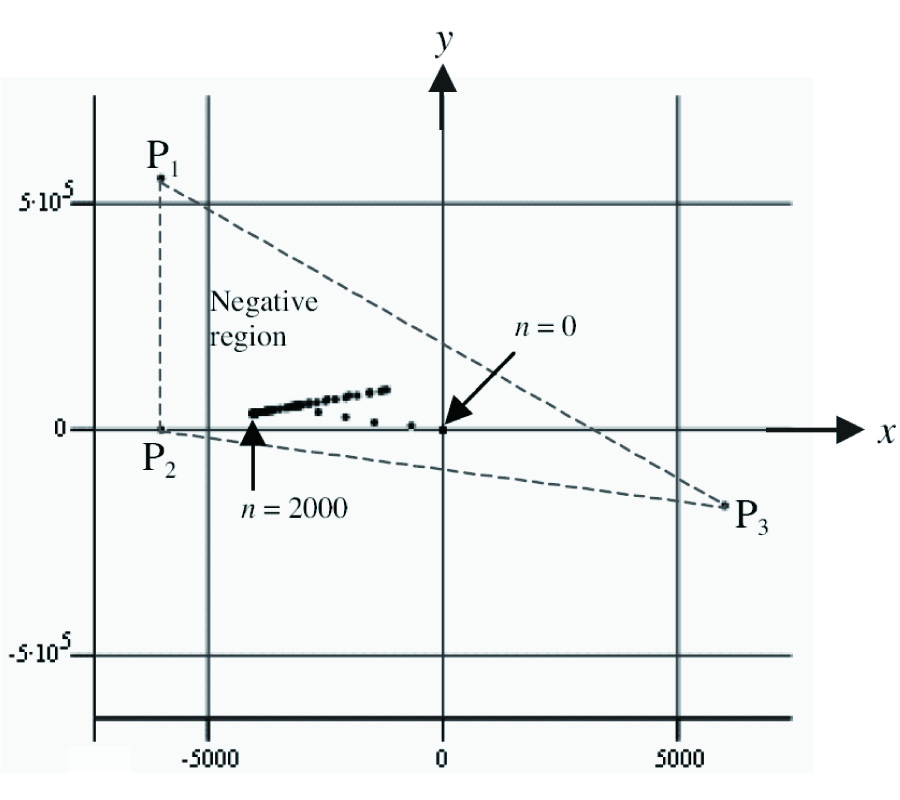# Vol. 42

Front:[PDF file] Back:[PDF file]
Latest Volume
All Volumes
All Issues

#### Stability of Classical Finite-Difference Time-Domain (FDTD) Formulation with Nonlinear Elements --- a New Perspective

By
Progress In Electromagnetics Research, Vol. 42, 49-89, 2003
doi:10.2528/PIER03010901

## Abstract

In this paper new stability theorems for Yee's Finite- Difference Time-Domain (FDTD) formulation are derived based on the energy method. A numerical energy expression is proposed. This numerical energy is dependent on the FDTD model's E and H field components. It is shown that if the numerical energy is bounded, then all the field components will also be bounded as the simulation proceeds. The theorems in this paper are inspired by similar results in nonlinear dynamical system. The new theorems are used to prove the stability of a FDTD model containing non-homogeneous dielectrics, perfect electric conductor (PEC) boundary, nonlinear dielectric and also linear/nonlinear lumped elements. The theorems are intended to complement the well-known Courant-Friedrich-Lewy (CFL) Criterion. Finally it is shown how the theorems can be used as a test, to determine if the formulation of new lumped element in FDTD is proper or not. A proper formulation will preserve the dynamical stability of the FDTD model. The finding reported in this paper will have implications in the manner stability analysis of FDTD algorithm is carried out in the future.

## Citation

, "Stability of Classical Finite-Difference Time-Domain (FDTD) Formulation with Nonlinear Elements --- a New Perspective," Progress In Electromagnetics Research, Vol. 42, 49-89, 2003.
doi:10.2528/PIER03010901
http://www.jpier.org/PIER/pier.php?paper=0301091

## References

1. Yee, K. S., "Numerical solution of initial boundary value problems involving Maxwell's equations in isotropic media," IEEE Trans. Antennas and Propagation, Vol. 14, No. 5, 302-307, 1966.

2. Piket-May, M. J., A. Taflove, and J. Baron, "FD-TD modeling of digital signal propagation in 3-D circuits with passive and active loads," IEEE Trans. Microwave Theoryand Techniques, Vol. 42, No. 8, 1514-1523, 1994.
doi:10.1109/22.297814

3. Taflove, A., Computational Electrodynamics â€” The finitedifference time-domain method, Artech House, 1995.

4. Kung, F. and H. T. Chuah, "Modeling a diode in FDTD," J. of Electromagnetic Waves and Appl., Vol. 16, No. 1, 99-110, 2002.

5. Kung, F. and H. T. Chuah, "Modeling of bipolar junction transistor in FDTD simulation of printed circuit board," Progress in Electromagnetic Research, Vol. 36, 179-192, 2002.
doi:10.2528/PIER02013001

6. Pereda, J. A., et al., "Analyzing the stability of the FDTD technique by combining the von Neumann method with the Routh-Hurwitz criterion," IEEE Trans. Microwave Theoryand Techniques, Vol. 49, No. 2, 377-381, 2001.
doi:10.1109/22.903100

7. Thiel, W. and L. P. B. Katehi, "Some aspects of stability and numerical dissipation of the finite-difference time-domain (FDTD) technique including passive and active lumped-elements," IEEE Trans. Microwave Theoryand Techniques, Vol. 50, No. 9, 2159-2165, 2002.
doi:10.1109/TMTT.2002.802330

8. Remis, R. F., "On the stability of the finite-difference time-domain method," J. of Computational Physics, Vol. 163, No. 1, 249-261, 2000.
doi:10.1006/jcph.2000.6573

9. Strikwerda, J. C., Finite Difference Schemes and Partial Differential Equations, Wadsworth & Brooks/Cole Mathematics Series, 1989.

10. Scheinerman, E. R., Invitation to Dynamical Systems, Prentice Hall, 1996.

11. Elaydi, S. N., Discrete Chaos, Chapman & Hall/CRC, 2000.

12. Merkin, D. R., Introduction to the Theoryof Stability, Springer- Verlag, 1997.

13. Khalil, H. K., Nonlinear Systems, 2nd edition, Prentice Hall, 1996.